﻿ 多状态空间信息网络拓扑生成优化算法Download PDF文章快速检索 高级检索

1. 大连大学 信息工程学院, 大连 116622;
2. 大连大学 通信与网络重点实验室, 大连 116622;
3. 南京理工大学 自动化学院, 南京 210094

Topological generation and optimization method in multi-state space information network
PAN Chengsheng1,2, XING Guixuan1,2, QI Yaowen3, YANG Li1,2
1. College of Information Engineering, Dalian University, Dalian 116622, China;
2. Communication and Networks Laboratory, Dalian University, Dalian 116622, China;
3. School of Automation, Nanjing University of Science and Technology, Nanjing 210094, China
Abstract: The topological generation and optimization of Space Information Network (SIN) in multi-state is studied based on the high dynamicity in SIN, the multi-state characteristics of satellites, and the requirements of communication time-delay and topological invulnerability. According to the periodicity of satellite constellation, a periodic table of satellite constellation topologies is established. Considering the constraints of satellite visibility and connectivity degree, a multi-objective optimization model of network topology is set up, with network average and maximal delay as optimization targets. An Improved Multi-Objective Simulated Annealing (IMOSA) algorithm is proposed and used for solving the structure of global optimization topology. Considering the multi-state characteristics, the time-delay of topology is optimized to satisfy the high dynamicity. The final simulation based on the Iridium Constellation with 66 Low Earth Orbit (LEO) satellites in multi-state situation shows that, the algorithm can maximize the decreasing of communication delay and obtain favorable invulnerability topology structures. Compared with the previous static typology, the communication ability of topology generated by the algorithm improved significantly.
Keywords: space information network    network topology    multi-objective optimization    node multi-state    multi-objective simulated annealing algorithm

1 卫星系统的多状态

2 卫星网络模型 2.1 卫星网络拓扑模型

 $\mathit{\boldsymbol{A}} = \left[ {\begin{array}{*{20}{c}} 0&{{a_{12}}}& \cdots &{{a_{1n}}}\\ {{a_{21}}}&0& \cdots &{{a_{2n}}}\\ \vdots & \vdots & \ddots & \vdots \\ {{a_{n1}}}&{{a_{n2}}}& \cdots &0 \end{array}} \right]$ （1）

 $\begin{array}{*{20}{c}} {{d_{\max}} = {{\left[ {{{\left( {{R_{\rm{d}}} + {H_i}} \right)}^2} - {{\left( {{R_{\rm{d}}}} \right)}^2}} \right]}^{\frac{1}{2}}} + }\\ {{{\left[ {{{\left( {{R_{\rm{d}}} + {H_j}} \right)}^2} - {{\left( {{R_{\rm{d}}}} \right)}^2}} \right]}^{\frac{1}{2}}}} \end{array}$ （2）

dij为2颗卫星之间的实际距离，其计算公式为

 ${d_{ij}} = \sqrt {{{\left( {{X_i} - {X_j}} \right)}^2} + {{\left( {{Y_i} - {Y_j}} \right)}^2} - {{\left( {{Z_i} - {Z_j}} \right)}^2}}$ （3）

 $\begin{array}{l} {X_ * } = H\left[ {\cos m\cos \left( {\mathit{\Omega } - {\mathit{\Omega }_{\rm{G}}}} \right) - } \right.\\ \;\;\;\;\;\left. {\sin m\sin\left( {\mathit{\Omega } - {\mathit{\Omega }_{\rm{G}}}} \right)\cos\alpha } \right] \end{array}$ （4）
 $\begin{array}{l} {Y_ * } = H\left[ {\cos m \sin\left( {\mathit{\Omega } - {\mathit{\Omega }_{\rm{G}}}} \right) - } \right.\\ \;\;\;\;\;\left. {\sin m\cos \left( {\mathit{\Omega } - {\mathit{\Omega }_{\rm{G}}}} \right)\cos\alpha } \right] \end{array}$ （5）
 ${Z_ * } = H\left( {\sin m\sin \alpha } \right)$ （6）
 $m = {m_{\rm{a}}} + {n_0}\left( {t - {t_0}} \right)$ （7）
 ${\mathit{\Omega }_{\rm{G}}} = {\omega _{\rm{e}}}\left( {t - {t_0}} \right)$ （8）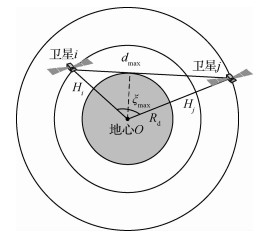图 1 卫星可视性判断示意图 Fig. 1 Judgment of satellites' visibility
2.2 拓扑周期模型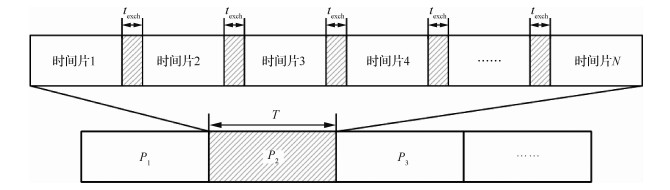图 2 时间片分割示意图 Fig. 2 Sketch map of time slots

3 卫星网络拓扑生成算法 3.1 算法建模

 $\min f\left( \mathit{\boldsymbol{A}} \right) = {\left[ {{f_{{\tau _a}}}\left( \mathit{\boldsymbol{A}} \right),{f_{{\tau _m}}}\left( \mathit{\boldsymbol{A}} \right)} \right]^{\rm{T}}}$ （9）

 ${\tau _{\rm{a}}} = \frac{1}{{n\left( {n - 1} \right)}}\sum\limits_{i = 1}^n {\sum\limits_{j = 1,j \ne i}^n {{c_{ij}}} }$ （10）
 ${\tau _{\rm{m}}} = \max {c_{ij}}$ （11）

 ${\rm{s}}.\;{\rm{t}}.\;\;\;{v_{ij}} \in \left\{ {0,1} \right\}$ （12）
 ${v_{ij}} = {v_{ji}}\;\;\;\;\;\;\forall i,j$ （13）
 ${v_{ij}} - {a_{ij}} \ge 0\;\;\;\;\;\;\forall i,j$ （14）
 $\sum\limits_{j = 1}^n {{a_{ij}}} \le k\;\;\;\;\;\forall i,j$ （15）
 ${c_{ij}} < \infty \;\;\forall i,j$ （16）

3.2 IMOSA算法

IMOSA算法流程如图 3所示。初始拓扑矩阵A0为待优化的静态拓扑矩阵，将其加入到Pareto解集中。每次迭代生成邻域解An，若新矩阵Anτaτm均优于前一次，则令其为新的当前矩阵An，每隔一定迭代次数从Pareto解集中随机选择一个矩阵作为初始矩阵，重新搜索；如果新矩阵未被接受，则保留当前矩阵进行下一次迭代。接受概率为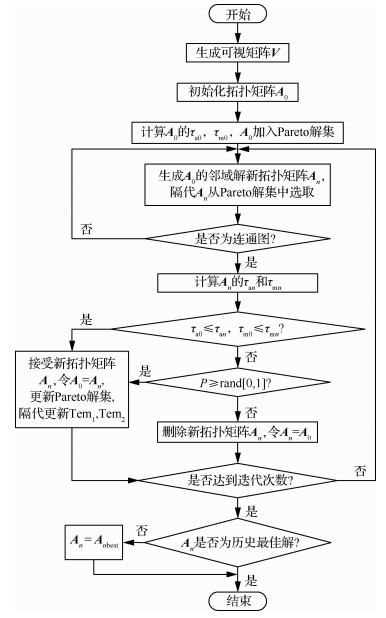图 3 IMOSA算法流程 Fig. 3 Process of IMOSA algorithm
 $P = \min \left\{ {1,\left[ {{\rm exp}\left( {\frac{{ - \Delta {\tau _{\rm{a}}}}}{{{\rm{Te}}{{\rm{m}}_1}}}} \right){\rm exp}\left( {\frac{{ - \varDelta {\tau _{\rm{m}}}}}{{{\rm{Te}}{{\rm{m}}_2}}}} \right)} \right]} \right\}$ （17）

3.3 算法复杂度

 $N\left[ {O\left( {{N^2}} \right) + O\left( E \right)} \right] = O\left( {{N^3}} \right)$ （18）

4 仿真分析 4.1 仿真环境

 卫星参数 数值 轨道高度/km 780 轨道倾角/(°) 86.3 轨道数目 6 轨道卫星数目 11 卫星总数 66 轨道面升交点赤经差/(°) 60 轨道周期/s 6 027.1 连接度 4

 卫星状态 概率分布/% 状态0 5 状态1 35 状态2 60
4.2 仿真结果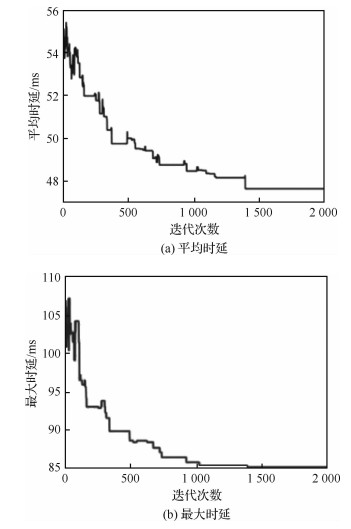图 4 单个时间片IMOSA算法收敛特性 Fig. 4 Convergence character of single time slot based on IMOSA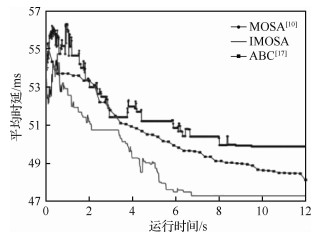图 5 IMOSA、MOSA和ABC算法收敛情况比较 Fig. 5 Comparison of convergence curves among IMOSA, MOSA and ABC algorithms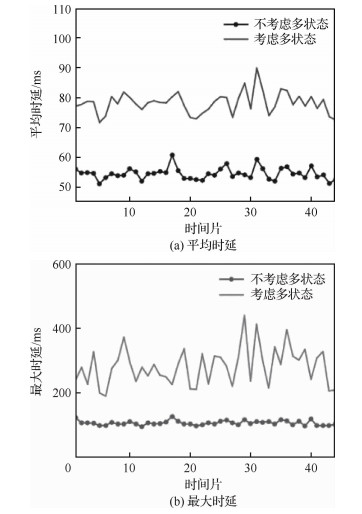图 6 铱星星座中考虑多状态因素时的网络时延改变 Fig. 6 Variation of network time delays considering multi-states in Iridium Constellation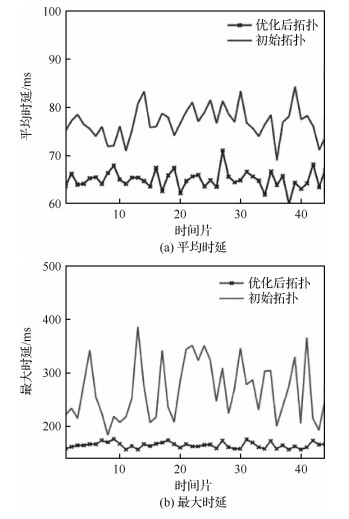图 7 铱星星座多状态下的IMOSA算法优化效果 Fig. 7 Results of optimization of IMOSA algorithm considering multi-states in Iridium Constellation

 $\bar \lambda = \ln \left( {\frac{1}{n}\sum\limits_{i = 1}^n {{{\rm{e}}^{{\lambda _i}}}} } \right)$ （19）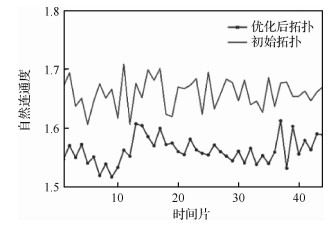图 8 IMOSA算法优化前后自然联通度对比 Fig. 8 Comparison of natural connectivity of whether using IMOSA algorithm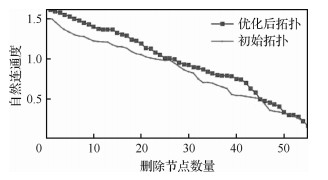图 9 单个时间片随机删除节点后自然联通度对比 Fig. 9 Comparison of natural connectivity between deleting nodes in single time-slot
5 结论

1) 该算法在铱星星座中收敛性较MOSA算法和ABC算法更好，与静态拓扑相比，网络端到端平均时延和最大时延分别最大降低16%和34%，实现了网络拓扑的优化。

2) 同时自然连通度的值提高了6%，即该算法生成的网络拓扑具有更好的抗毁性。

http://dx.doi.org/10.7527/S1000-6893.2019.23546

0

#### 文章信息

PAN Chengsheng, XING Guixuan, QI Yaowen, YANG Li

Topological generation and optimization method in multi-state space information network

Acta Aeronautica et Astronautica Sinica, 2020, 41(4): 323546.
http://dx.doi.org/10.7527/S1000-6893.2019.23546